## Solution to 1986 Problem 53

 The power radiated per unit solid angle for an oscillating electric dipole is proportional to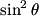$\sin^2 \theta$, where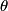$\theta$ is the angle between the electric dipole moment and point where the radiation is being measured. This implies that the radiated power per unit solid is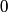$0$ along the$x$ axis and maximum on the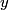$y$-$z$ plane. This implies that it is answer (A) or (C) that is correct. To determine whether the electric field is the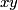$xy$-plane or in the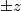$\pm z$ direction, recall that the electric field in the radiation zone of an oscillating electric dipole is in the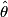$\hat{\theta}$ direction which in this case is in the$x$-$y$ plane. Therefore, answer (C) is correct.